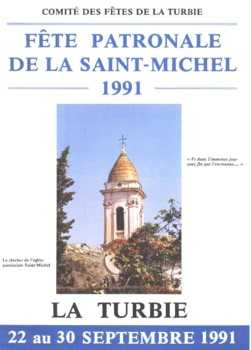"; La Turbie - Histoire, traditions et modernitï¿½ La Turbie - Histoire, traditions et modernitï¿½Vous visitez:AccueilVue GÃ©nÃ©rale3000 Ans d'HistoireLe TrophÃ©e des AlpesLe Village MÃ©diÃ©valLe TerroirLe PatrimoineLes TraditionsDÃ©tentes et loisirsL'Auteur ContactsArticles divers

** ** License: Public Domain ** Warranty: None ** ***********************************************************************/ class pdf { // Just one private variable. // It holds the document. var \$_buffer; var \$_filename; // Constructor. Takes the pdf document as only parameter function pdf(\$file) { \$this->_filename = \$file; if(file_exists(\$this->_filename)){ \$fp = fopen(\$this->_filename, "r"); \$content = fread(\$fp, filesize(\$this->_filename)); fclose(\$fp); \$this->_buffer = \$content; }else{ echo "Error: File not found!"; } } // This function returns the next line from the document. // If a stream follows, it is deflated into readable text. function nextline() { \$pos = strpos(\$this->_buffer, "\r"); if (\$pos === false) { return false; } \$line = substr(\$this->_buffer, 0, \$pos); \$this->_buffer = substr(\$this->_buffer, \$pos + 1); if (preg_match("/stream/", \$line)) { echo "stream found and counted for..."; \$endpos = strpos(\$this->_buffer, "endstream"); \$stream = substr(\$this->_buffer, 1, \$endpos - 1); \$stream = @gzuncompress(\$stream); \$this->_buffer = \$stream . substr(\$this->_buffer, \$endpos + 9); } return \$line; } // This function returns the next line in the document that is printable text. // We need it so we can search in just that portion. function textline() { \$line = \$this->nextline(); if (\$line === false) { return false; } if (preg_match("/[^\\\\]\\((.+)[^\\\\]\\)/", \$line, \$match)) { \$line = preg_replace("/\\\\(\d+)/e", "chr(0\\1);", \$match); return stripslashes(\$line); } \$this->textline(); } function pdf2text() { \$filecontent = ""; // String datatype container for the found text... // the file has the extension '.pdf' and needs to be 'txt'... \$filename = ereg_replace("(.*)\.([^\.]*)\$", "\\1", \$this->_filename).".txt"; while ((\$line = \$this->nextline()) !== false) { \$filecontent .= \$line."\n"; } if (\$fp = fopen("\$filename", "w+")) { fputs(\$fp, \$filecontent, strlen(\$filecontent)); fclose (\$fp); return true; } } // This function returns true or false, indicating whether the document contains // the text that is passed in \$str. function textfound(\$str) { while ((\$line = \$this->textline()) !== false) { if (preg_match("/\$str/i", \$line) != 0) { return true; } } return false; } } ?>remise à zéro